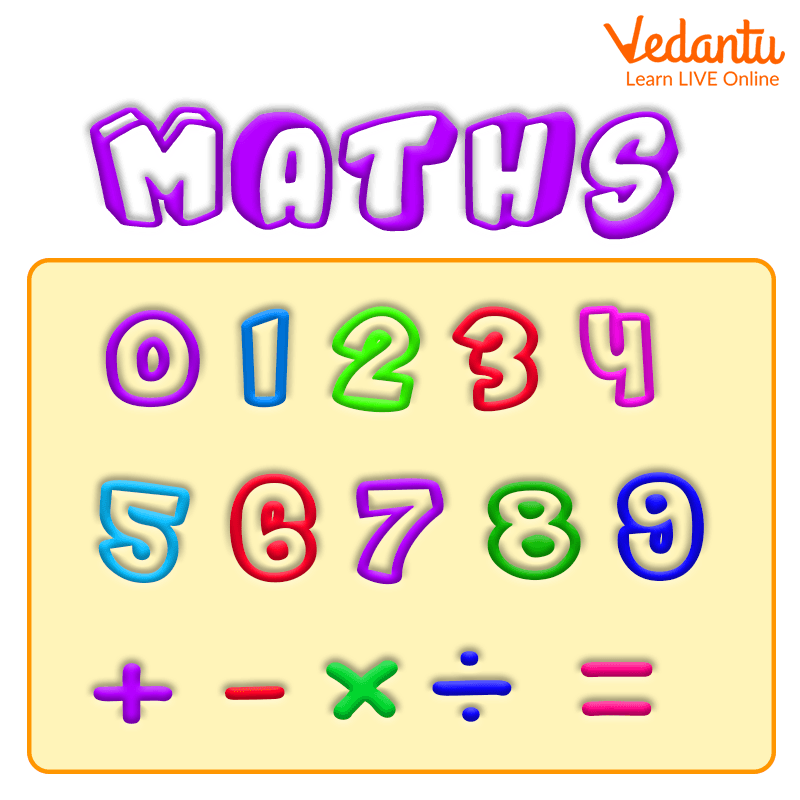Courses
Courses for Kids
Free study material
Free LIVE classes
More

# Syllabus of Maths for 10-Year OldsLIVE
Join Vedantu’s FREE Mastercalss

## An Introduction to New Concepts

As the kids get promoted to higher grades they are subjected to a lot of new concepts. They are taught new concepts and experiments in Science, Literature and Maths. Learning these new concepts can be fun and a little bit challenging especially for parents and teachers. But the challenge can be overcome with the proper orientation of the topics that are needed to be taught. This article deals with the introduction of concepts of Maths for 10-year-olds that are academically helpful. We aim to provide a list of topics that can be covered in the syllabus of Maths for 10-year-olds.Syllabus of maths for kids

## What Are the Topics That Are Covered in Maths for 10-year-olds?

The kids around the age of 10 or 11 are usually in Grade 5 or Grade 6. Needless to say, 10 and 11-year-olds are very young to be introduced to complex mathematical operations like trigonometry and calculus, but kids till this are familiarised with simple yet fascinating concepts like an introduction to number and number system, they might also have a brief idea about fractions. A 10-year old kid will use what they've learnt so far in arithmetic while also learning new abilities to help them answer more complex problems.

Kids in grade sixth are generally encouraged to work with numbers and learn about ratios. Kids are also taught how to convert measures, calculate volumes, and draw circles. The long division will be taught to them for dividing four-digit integers by two-digit numbers. A detailed list of topics that are covered in Maths for 10-year-olds is mentioned below.

• Number and place value

• Multiplication and division (introduction to long division)

• Fractions, decimals and percentages

• Geometry

• Measurement

• Statistics

• Ratio and proportion

• Introduction to algebra

Let us look briefly into the topics that can be taught in the above mentioned syllabus.

### Number and Place Value

Kids are taught about the number and number system. This can include the types of numbers like the whole number, integers, even and odd numbers. They are also taught about the use of place value charts.

Children will be expected to answer problems, particularly complex word problems with huge numbers, adding, subtracting, multiplying, and long division. This includes selecting efficient problem-solving strategies and double-checking their answers with a different method. Kids are also taught how to use brackets to explore the order of operations. Rounding answers to a defined degree of accuracy, such as to the nearest 10, 20, 50, and so on, is another common topic covered.

### Fractions, Decimals and Percentages

The concept of fractions, decimals and percentage calculation is one of the important concepts introduced to kids. The topic covered includes the study of addition, subtraction, division and multiplication of fractions. They are also introduced to different types of fractions and their simplification.

### Geometry

Kids are introduced to geometry in the syllabus of Maths for 10-year-olds. The geometry topics generally include an introduction to various shapes and structures. They are also taught to find the area of triangles, squares and circles.

### Statistics

Students are taught about presenting discrete and continuous data in a variety of ways in Statistics. To build and analyse pie charts, students must connect their work to angles, circles, fractions, and percentages. Students are also taught how to solve problems by understanding and creating line graphs, as well as how to calculate and interpret mean averages.

### Algebra

Students in Grade 6 are given a brief introduction to algebra. They are taught simple formulae of algebra. They are also taught about generating and describing linear number sequences and the use of letters to represent unknown numbers when solving number problems.

## Conclusion

This was the complete discussion on the topics that are covered in the syllabus of Maths for 10-year-olds. We hope to have helped parents and teachers to plan the study schedule accordingly to help kids better understand the concepts and excel academically. We request that all parents and teachers include real-life examples while teaching the concepts. This can help kids maintain their curiosity and appreciate the industrial and practical implementation of theories that they study.

Last updated date: 28th Sep 2023
Total views: 137.7k
Views today: 2.37k

## FAQs on Syllabus of Maths for 10-Year Olds

1. Mention an effective learning idea that parents can plan using everyday items at home.

Including activities and real-life examples while teaching a concept is always effective. Parents can use real-life scenarios that use large numbers like house prices and metre readings; this can help kids familiarise themselves with large numbers. Another effective way is to engage kids in developing recipes as they include various ratios and proportions, this can help kids understand the importance of such topics.

2. How to help kids in better understanding of Statistics?

Statistics is one of the important concepts introduced in the sixth grade. One of the ways to effectively teach the concept is to encourage kids to create line graphs and investigate the pie charts. Teachers can ask students to make a line graph on the temperature variation over a week. This can be a fun activity that can help kids with plotting a graph along with the presentation of continuous data.